### Rose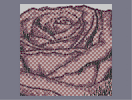Hover over the thumbnail for a full-size version.

Author espada777777 author:espada777777 featured flower-people rated 2009-10-21 2009-10-21 5 by 105 people. \$Rose#espada777777#flower-people#11111111111111111111111111111111111111111111111111111111111111111111111111111111111111111111000000000000000000000000000000000000000000000000000000000000000000000000000000000000000000000000000000000000000000000000000000000000000000000000000000000000000000000000000000000000000000000000000000000000000000000000000000000000000000000000000000000000000000000000000000000000000000000000000000000000000000000000000000000000000000000000000000000000000000000000000000000000000000000000000000000000000000000000000000000000000000000000000000000000000000000000000000000000000000000000000000000000000000000000000000000000000000000000011111111111111111111111111111111111111111111111111111111111111111111111111111111111111111111|5^610,102!5^611,104!12^420,36!12^432,36!12^456,36!12^468,36!12^480,36!12^492,36!12^504,36!12^504,36!12^516,36!12^192,48!12^204,48!12^252,48!12^264,48!12^276,48!12^288,48!12^300,48!12^312,48!12^324,48!12^336,48!12^348,48!12^360,48!12^372,48!12^324,60!12^312,60!12^300,60!12^288,60!12^264,60!12^276,60!12^252,60!12^240,60!12^228,60!12^216,60!12^204,60!12^192,60!12^180,60!12^168,60!12^144,72!12^156,72!12^168,72!12^180,72!12^192,72!12^204,72!12^216,72!12^228,72!12^240,72!12^252,72!12^264,72!12^276,72!12^288,72!12^264,84!12^252,84!12^240,84!12^228,84!12^216,84!12^204,84!12^192,84!12^180,84!12^168,84!12^156,84!12^144,84!12^132,84!12^120,96!12^132,96!12^144,96!12^156,96!12^168,96!12^180,96!12^192,96!12^204,96!12^216,96!12^228,96!12^240,96!12^252,96!12^240,108!12^216,108!12^228,108!12^204,108!12^192,108!12^180,108!12^168,108!12^156,108!12^144,108!12^132,108!12^120,108!12^120,120!12^132,120!12^132,120!12^144,120!12^156,120!12^168,120!12^180,120!12^192,120!12^204,120!12^216,120!12^228,120!12^228,132!12^216,132!12^204,132!12^192,132!12^180,132!12^156,132!12^168,132!12^144,132!12^132,132!12^120,132!12^120,144!12^132,144!12^144,144!12^156,144!12^168,144!12^180,144!12^192,144!12^204,144!12^216,144!12^216,156!12^216,168!12^204,180!12^204,192!12^204,204!12^204,216!12^192,228!12^192,240!12^192,252!12^204,156!12^192,156!12^180,156!12^168,156!12^156,156!12^144,156!12^132,156!12^120,156!12^120,168!12^132,168!12^144,168!12^156,168!12^168,168!12^180,168!12^192,168!12^204,168!12^192,180!12^192,192!12^192,216!12^192,204!12^180,180!12^168,180!12^156,180!12^144,180!12^132,180!12^120,180!12^120,192!12^120,204!12^120,216!12^120,228!12^120,240!12^120,252!12^120,264!12^132,264!12^144,264!12^156,264!12^168,264!12^180,264!12^180,252!12^180,240!12^180,228!12^180,216!12^180,204!12^180,192!12^168,192!12^168,204!12^168,216!12^168,228!12^168,228!12^168,240!12^168,252!12^156,252!12^144,252!12^132,252!12^132,240!12^132,228!12^132,216!12^132,204!12^132,192!12^144,192!12^144,204!12^144,216!12^144,240!12^144,228!12^156,192!12^156,204!12^156,216!12^156,228!12^156,240!12^180,276!12^180,288!12^180,300!12^180,312!12^180,324!12^180,336!12^180,348!12^180,360!12^180,372!12^192,372!12^192,384!12^192,396!12^192,408!12^192,420!12^204,420!12^204,432!12^204,444!12^204,456!12^216,456!12^216,468!12^228,480!12^240,492!12^252,504!12^264,504!12^240,504!12^228,504!12^228,504!12^228,492!12^216,504!12^216,492!12^216,480!12^204,480!12^204,468!12^192,468!12^192,456!12^192,444!12^192,432!12^180,432!12^180,420!12^180,408!12^180,396!12^180,384!12^168,384!12^168,372!12^168,360!12^168,348!12^168,336!12^168,324!12^168,312!12^168,300!12^168,288!12^168,276!12^156,276!12^156,288!12^156,300!12^156,312!12^156,324!12^156,336!12^156,348!12^156,360!12^144,348!12^144,336!12^144,312!12^144,324!12^144,300!12^144,288!12^144,276!12^132,336!12^120,336!12^132,324!12^120,324!12^120,312!12^132,312!12^132,300!12^120,300!12^120,288!12^132,288!12^132,276!12^120,276!12^132,348!12^120,348!12^120,360!12^132,360!12^132,372!12^120,372!12^120,384!12^132,384!12^144,384!12^144,372!12^144,360!12^156,372!12^156,384!12^156,396!12^168,396!12^168,408!12^168,420!12^168,432!12^168,444!12^168,456!12^168,468!12^168,492!12^168,480!12^180,492!12^180,480!12^180,468!12^180,456!12^180,444!12^192,480!12^192,492!12^192,504!12^204,504!12^204,480!12^204,492!12^156,480!12^156,468!12^156,456!12^156,444!12^156,432!12^156,420!12^156,408!12^144,396!12^144,408!12^144,420!12^144,432!12^144,444!12^144,456!12^144,468!12^144,480!12^144,492!12^132,492!12^132,480!12^132,456!12^132,468!12^132,444!12^132,432!12^132,420!12^132,408!12^132,396!12^120,396!12^120,408!12^120,420!12^120,432!12^120,444!12^120,468!12^120,456!12^120,480!12^120,492!12^120,504!12^132,504!12^132,516!12^120,516!12^120,528!12^120,540!12^120,552!12^120,564!12^120,576!12^132,552!12^132,564!12^132,576!12^144,576!12^144,564!12^156,564!12^156,576!12^168,576!12^180,576!12^192,576!12^204,576!12^216,576!12^228,576!12^240,576!12^252,576!12^264,576!12^276,576!12^288,576!12^156,564!12^168,564!12^180,564!12^180,552!12^168,552!12^156,552!12^144,552!12^132,540!12^132,528!12^144,540!12^144,528!12^144,516!12^144,504!12^156,492!12^156,504!12^156,516!12^156,528!12^156,540!12^168,540!12^168,528!12^168,516!12^168,504!12^180,504!12^180,516!12^180,528!12^180,540!12^192,564!12^192,552!12^192,540!12^192,528!12^192,516!12^204,516!12^204,528!12^204,540!12^204,552!12^204,552!12^204,564!12^216,564!12^216,552!12^216,540!12^216,528!12^216,516!12^228,516!12^228,528!12^228,540!12^228,552!12^228,564!12^240,564!12^240,552!12^240,540!12^240,528!12^240,516!12^252,516!12^252,540!12^252,528!12^252,552!12^252,564!12^264,564!12^264,552!12^264,540!12^264,528!12^264,516!12^276,516!12^276,528!12^276,540!12^276,552!12^276,564!12^288,564!12^288,540!12^288,552!12^288,528!12^288,516!12^300,516!12^300,528!12^300,540!12^300,552!12^300,564!12^300,564!12^300,576!12^312,576!12^312,564!12^312,552!12^312,540!12^312,528!12^324,528!12^336,528!12^348,528!12^360,516!12^372,516!12^384,516!12^396,504!12^408,504!12^420,492!12^432,480!12^444,468!12^444,456!12^456,444!12^456,432!12^456,420!12^324,540!12^324,552!12^324,564!12^324,576!12^336,576!12^336,564!12^336,552!12^348,540!12^336,540!12^348,552!12^348,564!12^348,576!12^360,576!12^360,564!12^360,552!12^360,540!12^360,528!12^372,528!12^372,540!12^372,552!12^372,564!12^372,576!12^384,576!12^384,564!12^384,552!12^384,540!12^384,528!12^396,516!12^396,528!12^396,540!12^396,552!12^396,564!12^396,576!12^408,576!12^408,564!12^408,540!12^408,552!12^408,528!12^408,516!12^420,504!12^420,516!12^420,540!12^420,528!12^420,552!12^420,564!12^420,576!12^432,576!12^432,564!12^432,552!12^432,528!12^432,540!12^432,516!12^432,504!12^432,492!12^444,480!12^444,492!12^444,504!12^444,516!12^444,528!12^444,540!12^444,540!12^444,552!12^444,564!12^444,576!12^456,576!12^456,564!12^456,552!12^456,540!12^456,528!12^456,516!12^456,504!12^456,492!12^456,480!12^456,468!12^456,456!12^468,408!12^468,396!12^468,384!12^456,372!12^456,360!12^456,348!12^468,420!12^468,432!12^468,444!12^468,456!12^468,468!12^468,480!12^468,492!12^468,504!12^468,516!12^468,540!12^468,528!12^468,552!12^468,564!12^468,576!12^468,372!12^468,360!12^468,348!12^468,336!12^444,336!12^444,324!12^456,336!12^456,324!12^468,324!12^432,312!12^444,312!12^456,312!12^468,312!12^420,300!12^408,300!12^432,300!12^444,300!12^456,300!12^468,300!12^396,288!12^384,288!12^372,288!12^360,300!12^348,300!12^348,288!12^360,288!12^408,288!12^432,288!12^420,288!12^444,288!12^456,288!12^468,288!12^336,312!12^336,300!12^372,276!12^384,276!12^396,276!12^408,276!12^420,276!12^432,276!12^444,276!12^456,276!12^432,264!12^408,264!12^396,264!12^420,264!12^324,312!12^324,324!12^324,336!12^312,324!12^312,336!12^312,348!12^312,360!12^312,372!12^312,384!12^312,396!12^480,576!12^480,564!12^480,552!12^480,540!12^480,528!12^480,516!12^480,504!12^480,492!12^480,480!12^480,468!12^480,456!12^480,444!12^492,432!12^480,432!12^480,420!12^480,408!12^480,396!12^480,384!12^480,372!12^480,360!12^480,348!12^480,336!12^480,324!12^480,312!12^480,300!12^492,312!12^492,324!12^492,336!12^492,348!12^492,360!12^492,372!12^492,384!12^492,396!12^492,408!12^492,420!12^504,324!12^516,324!12^504,336!12^516,336!12^504,348!12^516,348!12^516,360!12^504,360!12^504,372!12^516,372!12^516,384!12^504,384!12^504,408!12^504,396!12^516,396!12^516,408!12^504,420!12^492,444!12^492,468!12^492,456!12^492,480!12^492,492!12^492,504!12^492,528!12^492,516!12^504,540!12^492,540!12^492,552!12^492,564!12^492,576!12^504,576!12^504,564!12^504,552!12^516,540!12^516,552!12^516,564!12^516,576!12^528,576!12^528,564!12^528,552!12^528,540!12^504,528!12^504,516!12^504,504!12^504,492!12^504,480!12^504,468!12^504,456!12^504,444!12^504,432!12^516,420!12^516,432!12^516,444!12^516,456!12^516,468!12^516,480!12^516,492!12^516,504!12^516,528!12^516,516!12^528,528!12^528,516!12^528,504!12^528,492!12^528,480!12^528,468!12^528,456!12^528,444!12^528,432!12^528,420!12^528,408!12^528,396!12^528,384!12^528,372!12^528,360!12^528,348!12^528,336!12^528,336!12^540,336!12^552,336!12^540,348!12^540,360!12^540,372!12^540,384!12^540,396!12^540,408!12^540,420!12^540,432!12^540,444!12^540,456!12^540,468!12^540,480!12^540,492!12^540,504!12^540,516!12^540,528!12^540,540!12^540,564!12^540,552!12^540,576!12^552,576!12^552,564!12^552,552!12^552,540!12^552,528!12^552,516!12^552,504!12^552,492!12^552,480!12^552,468!12^552,456!12^552,444!12^552,432!12^552,420!12^552,408!12^552,396!12^552,384!12^552,372!12^552,360!12^552,348!12^564,336!12^564,348!12^564,360!12^564,372!12^564,384!12^564,396!12^564,396!12^564,408!12^564,432!12^564,420!12^564,444!12^564,456!12^564,468!12^564,480!12^564,504!12^564,492!12^564,516!12^564,528!12^576,540!12^564,540!12^564,552!12^564,564!12^564,576!12^576,576!12^576,564!12^588,552!12^576,552!12^576,528!12^576,516!12^576,504!12^576,492!12^588,564!12^588,576!12^600,576!12^600,564!12^600,552!12^588,540!12^588,528!12^588,516!12^588,504!12^588,492!12^576,480!12^576,456!12^576,468!12^576,432!12^576,444!12^576,420!12^576,396!12^576,408!12^576,384!12^576,372!12^576,360!12^576,336!12^576,348!12^576,324!12^588,324!12^600,312!12^588,336!12^588,348!12^588,360!12^588,372!12^588,384!12^588,396!12^588,408!12^588,420!12^588,432!12^588,456!12^588,444!12^588,468!12^588,480!12^600,540!12^600,528!12^600,516!12^600,492!12^600,504!12^600,480!12^600,468!12^600,456!12^600,444!12^600,432!12^600,420!12^600,408!12^600,396!12^600,384!12^600,372!12^600,360!12^600,348!12^600,336!12^600,324!12^612,300!12^624,288!12^624,276!12^636,264!12^636,252!12^636,240!12^648,228!12^648,216!12^648,204!12^648,192!12^648,180!12^636,168!12^636,156!12^636,144!12^648,240!12^648,252!12^648,264!12^648,276!12^636,276!12^636,288!12^648,288!12^648,300!12^636,300!12^624,300!12^612,312!12^624,312!12^636,312!12^648,312!12^636,324!12^624,324!12^612,324!12^612,336!12^612,348!12^612,360!12^612,372!12^612,384!12^612,396!12^612,408!12^612,420!12^612,432!12^612,444!12^612,456!12^612,468!12^612,480!12^612,492!12^612,504!12^612,516!12^612,528!12^612,540!12^612,552!12^612,564!12^612,576!12^624,576!12^624,564!12^624,552!12^636,540!12^624,540!12^624,528!12^624,516!12^624,504!12^624,492!12^624,480!12^624,468!12^624,456!12^624,444!12^624,432!12^624,420!12^624,408!12^624,396!12^624,384!12^624,372!12^624,360!12^624,336!12^624,348!12^636,336!12^636,360!12^636,348!12^636,372!12^636,384!12^636,396!12^636,408!12^636,420!12^636,432!12^636,444!12^636,468!12^648,456!12^636,456!12^636,480!12^636,492!12^636,504!12^636,528!12^636,516!12^636,552!12^636,564!12^636,576!12^648,576!12^648,564!12^648,540!12^648,552!12^648,528!12^648,516!12^648,492!12^648,504!12^648,480!12^648,468!12^660,576!12^672,576!12^672,564!12^660,564!12^672,552!12^660,552!12^660,540!12^672,540!12^672,528!12^660,528!12^660,516!12^672,516!12^672,504!12^660,504!12^660,492!12^672,492!12^672,480!12^660,480!12^660,468!12^672,468!12^672,456!12^660,456!12^648,444!12^648,432!12^648,420!12^648,408!12^648,396!12^648,384!12^648,372!12^648,360!12^648,348!12^648,336!12^648,324!12^660,444!12^672,444!12^672,432!12^660,432!12^660,420!12^672,420!12^672,408!12^660,408!12^660,396!12^672,396!12^672,384!12^660,384!12^660,372!12^660,372!12^672,372!12^672,348!12^672,360!12^660,360!12^660,348!12^660,336!12^660,312!12^660,300!12^672,300!12^672,312!12^672,324!12^672,336!12^660,288!12^672,276!12^660,276!12^672,288!12^672,264!12^660,264!12^660,252!12^672,240!12^672,252!12^660,240!12^660,228!12^672,228!12^672,216!12^660,216!12^660,204!12^672,204!12^672,192!12^660,192!12^660,180!12^672,180!12^648,168!12^660,168!12^672,168!12^672,156!12^648,156!12^660,156!12^648,144!12^660,144!12^672,144!12^624,132!12^624,120!12^612,108!12^600,96!12^588,84!12^576,72!12^564,72!12^552,60!12^540,60!12^528,60!12^516,48!12^504,48!12^492,48!12^468,48!12^480,48!12^528,48!12^540,48!12^552,48!12^564,60!12^576,60!12^588,60!12^588,72!12^600,84!12^600,72!12^600,60!12^612,72!12^612,84!12^612,96!12^624,108!12^624,96!12^624,84!12^624,72!12^636,72!12^636,84!12^636,96!12^636,108!12^636,120!12^636,132!12^660,132!12^648,132!12^648,120!12^648,108!12^648,108!12^648,96!12^648,72!12^648,84!12^648,60!12^660,60!12^660,72!12^660,72!12^660,84!12^660,96!12^660,108!12^660,120!12^672,132!12^672,120!12^672,108!12^672,96!12^672,84!12^672,72!12^312,408!12^312,432!12^312,420!12^312,444!12^312,456!12^312,468!12^312,480!12^312,492!12^312,504!12^312,516!12^324,516!12^324,492!12^324,504!12^336,516!12^348,516!12^336,504!12^348,504!12^372,504!12^384,504!12^360,504!12^360,492!12^348,492!12^336,492!12^324,480!12^336,480!12^348,480!12^336,468!12^324,468!12^336,456!12^324,456!12^324,444!12^324,432!12^324,420!12^324,408!12^324,396!12^324,384!12^324,372!12^324,360!12^324,348!12^336,336!12^336,324!12^348,312!12^360,312!12^372,300!12^384,300!12^396,300!12^408,312!12^420,312!12^420,324!12^432,324!12^432,348!12^432,336!12^444,348!12^444,360!12^444,372!12^456,384!12^456,396!12^456,408!12^444,432!12^444,444!12^444,420!12^444,408!12^444,396!12^444,384!12^432,360!12^432,372!12^432,396!12^432,384!12^432,408!12^432,420!12^432,432!12^432,444!12^432,456!12^432,468!12^420,480!12^420,468!12^420,456!12^420,444!12^420,432!12^420,420!12^420,408!12^420,396!12^420,372!12^420,360!12^420,348!12^420,336!12^420,384!12^372,312!12^384,312!12^396,312!12^408,324!12^384,324!12^396,324!12^372,324!12^360,324!12^348,324!12^348,336!12^360,336!12^372,336!12^384,336!12^396,336!12^408,336!12^336,348!12^336,360!12^336,372!12^336,384!12^336,396!12^336,408!12^336,420!12^336,444!12^336,432!12^348,468!12^348,456!12^348,444!12^348,432!12^348,420!12^348,408!12^348,396!12^348,384!12^348,372!12^348,360!12^348,348!12^360,348!12^360,360!12^360,384!12^360,372!12^372,360!12^372,348!12^384,348!12^384,360!12^396,360!12^396,348!12^408,348!12^408,360!12^408,384!12^408,372!12^408,396!12^408,408!12^408,420!12^408,432!12^408,444!12^408,456!12^408,468!12^408,480!12^408,492!12^396,492!12^384,492!12^372,492!12^360,480!12^360,468!12^360,456!12^360,444!12^360,432!12^360,420!12^360,408!12^360,396!12^372,372!12^384,372!12^396,372!12^396,396!12^396,372!12^396,384!12^396,408!12^396,420!12^396,420!12^396,432!12^396,444!12^396,456!12^396,468!12^396,480!12^384,480!12^372,480!12^372,468!12^372,456!12^372,444!12^372,420!12^372,432!12^372,408!12^372,396!12^372,384!12^384,384!12^384,396!12^384,408!12^384,420!12^384,432!12^384,444!12^384,456!12^384,468!12^300,336!12^300,348!12^300,360!12^288,360!12^288,384!12^288,372!12^288,396!12^276,384!12^276,396!12^276,408!12^276,420!12^276,432!12^264,408!12^264,420!12^264,432!12^276,444!12^276,456!12^288,456!12^288,468!12^288,480!12^300,492!12^276,504!12^300,480!12^300,468!12^300,456!12^288,444!12^288,432!12^288,420!12^288,408!12^300,372!12^300,384!12^300,396!12^300,420!12^300,408!12^300,432!12^300,444!12^300,504!12^288,504!12^288,492!12^276,492!12^264,492!12^252,492!12^276,480!12^264,480!12^252,480!12^240,480!12^276,468!12^264,468!12^252,468!12^240,468!12^228,468!12^228,456!12^240,456!12^252,456!12^264,456!12^264,444!12^252,444!12^240,444!12^228,444!12^216,444!12^216,432!12^240,432!12^228,432!12^252,432!12^252,420!12^240,420!12^228,420!12^216,420!12^204,408!12^216,408!12^228,408!12^240,408!12^252,408!12^264,396!12^240,396!12^252,396!12^228,396!12^216,396!12^204,396!12^204,384!12^216,384!12^228,384!12^240,384!12^252,384!12^264,384!12^276,372!12^264,372!12^252,372!12^240,372!12^228,372!12^216,372!12^204,372!12^192,360!12^204,360!12^216,360!12^228,360!12^240,360!12^252,360!12^264,360!12^276,360!12^288,348!12^276,348!12^264,348!12^240,348!12^252,348!12^228,348!12^216,348!12^204,348!12^192,348!12^192,336!12^216,336!12^204,336!12^228,336!12^240,336!12^252,336!12^264,336!12^276,336!12^288,336!12^300,324!12^288,324!12^276,324!12^264,324!12^252,324!12^240,324!12^228,324!12^216,324!12^204,324!12^192,324!12^192,312!12^204,312!12^216,312!12^240,312!12^228,312!12^252,312!12^264,312!12^276,312!12^288,312!12^312,312!12^300,312!12^324,300!12^312,300!12^300,300!12^288,300!12^276,300!12^264,300!12^252,300!12^240,300!12^228,300!12^216,300!12^192,300!12^204,300!12^192,288!12^204,288!12^216,288!12^228,288!12^240,288!12^252,288!12^276,288!12^264,288!12^288,288!12^300,288!12^312,288!12^324,288!12^336,288!12^360,276!12^348,276!12^336,276!12^324,276!12^312,276!12^300,276!12^288,276!12^276,276!12^264,276!12^252,276!12^240,276!12^228,276!12^216,276!12^204,276!12^192,276!12^192,264!12^204,264!12^228,264!12^216,264!12^240,264!12^252,264!12^264,264!12^288,264!12^276,264!12^300,264!12^312,264!12^324,264!12^336,264!12^348,264!12^360,264!12^372,264!12^384,264!12^360,252!12^336,252!12^348,252!12^324,252!12^300,252!12^312,252!12^288,252!12^276,252!12^264,252!12^252,252!12^240,252!12^240,252!12^228,252!12^216,252!12^204,252!12^204,240!12^216,240!12^240,240!12^228,240!12^252,240!12^264,240!12^276,240!12^288,240!12^300,240!12^324,240!12^312,240!12^204,228!12^216,228!12^228,228!12^240,228!12^264,228!12^252,228!12^276,228!12^288,228!12^300,228!12^276,216!12^264,216!12^252,216!12^240,216!12^228,216!12^216,216!12^216,204!12^216,192!12^216,180!12^228,180!12^228,192!12^228,204!12^240,204!12^240,192!12^372,252!12^360,240!12^348,240!12^336,240!12^348,228!12^336,228!12^324,228!12^312,228!12^336,216!12^324,216!12^312,216!12^300,216!12^288,216!12^324,204!12^312,204!12^300,204!12^288,204!12^276,204!12^264,204!12^252,204!12^252,192!12^264,192!12^276,192!12^288,192!12^312,192!12^300,192!12^300,180!12^288,180!12^276,180!12^252,180!12^240,180!12^264,180!12^288,168!12^276,168!12^264,168!12^252,168!12^240,168!12^228,168!12^228,156!12^252,156!12^240,156!12^264,156!12^276,156!12^264,144!12^252,144!12^240,144!12^228,144!12^240,132!12^252,132!12^240,120!12^384,252!12^372,240!12^360,228!12^348,216!12^336,204!12^336,192!12^324,192!12^324,180!12^312,180!12^312,168!12^300,168!12^300,156!12^288,156!12^288,144!12^276,144!12^276,132!12^264,132!12^264,120!12^252,120!12^276,120!12^264,108!12^252,108!12^264,96!12^384,240!12^372,228!12^360,216!12^348,204!12^348,192!12^336,180!12^336,168!12^324,168!12^324,156!12^312,156!12^312,144!12^300,144!12^300,132!12^300,120!12^288,132!12^288,120!12^288,108!12^288,96!12^276,108!12^276,96!12^276,84!12^288,84!12^396,252!12^384,228!12^372,216!12^372,204!12^360,204!12^360,192!12^360,180!12^348,180!12^348,168!12^348,156!12^336,156!12^336,144!12^336,132!12^336,120!12^324,144!12^324,132!12^312,132!12^312,120!12^324,120!12^324,108!12^312,108!12^300,108!12^300,96!12^324,96!12^324,84!12^324,72!12^312,96!12^312,84!12^312,72!12^300,72!12^300,84!12^396,240!12^384,216!12^384,204!12^372,192!12^372,180!12^360,168!12^360,156!12^360,144!12^348,144!12^348,132!12^348,120!12^348,108!12^336,108!12^336,96!12^336,84!12^336,72!12^336,60!12^396,228!12^384,192!12^384,180!12^372,168!12^372,156!12^372,144!12^372,132!12^360,132!12^360,120!12^360,108!12^360,96!12^360,84!12^348,96!12^348,84!12^348,60!12^348,72!12^396,216!12^396,204!12^384,168!12^384,156!12^384,144!12^372,120!12^372,108!12^372,96!12^372,84!12^360,72!12^360,60!12^396,192!12^396,180!12^396,168!12^384,132!12^384,120!12^384,108!12^384,96!12^372,72!12^372,60!12^396,156!12^396,144!12^396,132!12^396,120!12^384,84!12^384,72!12^384,60!12^384,48!12^396,108!12^396,96!12^396,72!12^396,84!12^396,60!12^396,48!12^408,144!12^408,132!12^408,108!12^408,120!12^408,96!12^408,84!12^408,72!12^408,60!12^408,48!12^420,48!12^420,60!12^420,72!12^420,84!12^420,96!12^420,108!12^420,120!12^432,72!12^432,60!12^432,48!12^408,156!12^408,168!12^408,192!12^408,180!12^408,204!12^408,228!12^408,204!12^408,216!12^420,192!12^420,180!12^420,168!12^420,156!12^420,144!12^420,132!12^432,156!12^432,144!12^432,132!12^432,120!12^432,108!12^432,84!12^432,96!12^444,120!12^444,108!12^444,84!12^444,96!12^444,72!12^444,60!12^444,48!12^456,48!12^456,60!12^456,72!12^456,84!12^456,96!12^468,84!12^468,72!12^468,60!12^480,72!12^480,60!12^492,60!12^408,240!12^420,216!12^420,204!12^432,192!12^432,180!12^432,168!12^444,168!12^444,156!12^444,144!12^444,132!12^456,144!12^456,132!12^456,120!12^456,108!12^468,120!12^468,108!12^468,96!12^480,96!12^480,84!12^492,84!12^492,72!12^504,72!12^504,60!12^516,60!12^420,228!12^432,216!12^432,204!12^444,204!12^444,192!12^444,180!12^456,192!12^456,180!12^456,168!12^456,156!12^468,180!12^468,168!12^468,156!12^468,144!12^468,132!12^480,168!12^480,156!12^480,144!12^480,132!12^480,120!12^480,108!12^492,156!12^492,144!12^492,132!12^492,108!12^492,120!12^492,96!12^504,144!12^504,132!12^504,120!12^504,108!12^504,96!12^504,84!12^516,132!12^516,120!12^516,108!12^516,96!12^516,84!12^516,72!12^528,120!12^528,108!12^528,96!12^528,84!12^528,72!12^540,108!12^540,96!12^540,84!12^540,72!12^552,96!12^552,84!12^552,72!12^564,84!12^492,168!12^504,156!12^516,156!12^516,144!12^528,144!12^528,132!12^540,132!12^540,120!12^564,120!12^552,120!12^552,108!12^564,108!12^576,108!12^564,96!12^576,96!12^576,84!12^588,96!12^588,108!12^600,108!12^612,120!12^600,120!12^588,120!12^576,120!12^588,132!12^576,132!12^552,132!12^564,132!12^564,144!12^552,144!12^540,144!12^552,156!12^540,156!12^528,156!12^528,168!12^516,168!12^504,168!12^504,180!12^492,180!12^480,180!12^480,192!12^468,192!12^468,204!12^456,204!12^456,216!12^444,216!12^432,228!12^600,132!12^612,132!12^624,144!12^612,144!12^600,144!12^588,144!12^576,144!12^600,156!12^588,156!12^576,156!12^564,156!12^576,168!12^564,168!12^552,168!12^540,168!12^552,180!12^540,180!12^528,180!12^516,180!12^528,192!12^516,192!12^504,192!12^492,192!12^504,204!12^504,204!12^492,204!12^480,204!12^564,180!12^576,180!12^588,168!12^600,168!12^612,168!12^624,168!12^624,156!12^612,156!12^420,240!12^444,228!12^456,228!12^468,228!12^468,216!12^480,216!12^492,216!12^504,216!12^516,216!12^528,216!12^540,216!12^516,204!12^528,204!12^540,204!12^552,204!12^564,204!12^576,204!12^588,204!12^600,204!12^612,204!12^540,192!12^552,192!12^576,192!12^564,192!12^588,192!12^612,192!12^600,192!12^624,192!12^636,192!12^636,180!12^624,180!12^600,180!12^588,180!12^612,180!12^420,240!12^432,240!12^444,240!12^456,240!12^468,240!12^480,228!12^492,228!12^504,228!12^516,228!12^528,228!12^540,228!12^552,228!12^564,228!12^576,228!12^588,228!12^588,216!12^600,216!12^612,216!12^624,216!12^636,216!12^636,204!12^624,204!12^576,216!12^564,216!12^540,216!12^552,216!12^600,228!12^624,228!12^612,228!12^636,228!12^624,240!12^612,240!12^600,240!12^588,240!12^564,240!12^576,240!12^408,252!12^420,252!12^432,252!12^444,252!12^456,252!12^468,252!12^492,252!12^480,252!12^480,240!12^492,240!12^504,240!12^516,240!12^528,240!12^540,240!12^552,240!12^624,252!12^612,252!12^624,264!12^612,264!12^612,276!12^600,264!12^588,264!12^564,264!12^540,264!12^516,264!12^492,264!12^468,264!12^456,264!12^444,264!12^480,264!12^504,264!12^504,252!12^516,252!12^528,252!12^540,252!12^552,252!12^564,252!12^576,252!12^588,252!12^600,252!12^504,276!12^504,288!12^504,300!12^504,312!12^516,288!12^528,288!12^528,276!12^528,264!12^552,264!12^552,276!12^552,288!12^552,300!12^552,312!12^564,312!12^576,312!12^576,300!12^576,288!12^576,276!12^576,264!12^600,276!12^600,288!12^600,300!12^564,324!12^552,324!12^552,324!12^540,324!12^528,324!12^480,288!12^480,276!12^468,276!12^492,300!12^492,288!12^492,276!12^516,276!12^516,300!12^516,312!12^528,312!12^528,300!12^540,312!12^540,300!12^540,288!12^540,276!12^564,276!12^564,288!12^564,300!12^588,312!12^588,300!12^588,288!12^588,276!12^612,288!12^522,42!12^498,42!12^474,42!12^450,42!12^426,42!12^402,42!12^378,42!12^354,42!12^282,42!12^174,54!12^198,54!12^222,54!12^246,54!12^270,54!12^294,54!12^318,54!12^342,54!12^366,54!12^390,54!12^414,54!12^438,54!12^462,54!12^486,54!12^510,54!12^534,54!12^558,54!12^582,54!12^666,66!12^642,66!12^618,66!12^594,66!12^570,66!12^546,66!12^522,66!12^498,66!12^474,66!12^450,66!12^426,66!12^402,66!12^378,66!12^354,66!12^330,66!12^306,66!12^282,66!12^258,66!12^234,66!12^210,66!12^186,66!12^162,66!12^150,78!12^174,78!12^198,78!12^222,78!12^246,78!12^270,78!12^294,78!12^318,78!12^342,78!12^366,78!12^390,78!12^414,78!12^378,90!12^354,90!12^330,90!12^306,90!12^282,90!12^258,90!12^234,90!12^210,90!12^186,90!12^162,90!12^138,90!12^126,102!12^150,102!12^174,102!12^198,102!12^222,102!12^246,102!12^270,102!12^294,102!12^318,102!12^306,114!12^282,114!12^258,114!12^234,114!12^210,114!12^186,114!12^162,114!12^138,114!12^126,126!12^150,126!12^174,126!12^198,126!12^222,126!12^246,126!12^270,126!12^294,126!12^282,138!12^258,138!12^234,138!12^210,138!12^186,138!12^162,138!12^138,138!12^126,150!12^150,150!12^174,150!12^198,150!12^222,150!12^246,150!12^270,150!12^258,162!12^246,174!12^234,186!12^222,198!12^222,222!12^210,234!12^210,210!12^234,162!12^210,162!12^186,162!12^162,162!12^138,162!12^126,174!12^150,174!12^174,174!12^198,174!12^222,174!12^210,186!12^186,186!12^162,186!12^138,186!12^126,198!12^150,198!12^198,198!12^174,198!12^186,210!12^162,210!12^138,210!12^126,222!12^126,246!12^126,270!12^126,294!12^138,234!12^138,258!12^138,282!12^138,306!12^126,318!12^138,330!12^126,342!12^138,354!12^126,366!12^138,378!12^126,390!12^138,402!12^126,414!12^138,426!12^126,438!12^138,450!12^126,462!12^138,474!12^126,486!12^138,498!12^126,510!12^138,522!12^126,534!12^138,546!12^126,558!12^138,570!12^198,222!12^174,222!12^150,222!12^150,246!12^150,270!12^150,294!12^150,318!12^150,342!12^150,366!12^150,390!12^150,414!12^150,438!12^150,462!12^150,486!12^150,510!12^150,534!12^150,558!12^162,570!12^162,546!12^162,522!12^162,498!12^174,510!12^174,534!12^174,558!12^186,570!12^186,546!12^186,522!12^210,522!12^198,534!12^210,546!12^198,558!12^210,570!12^222,558!12^234,570!12^234,546!12^222,534!12^246,534!12^234,522!12^258,522!12^282,522!12^306,522!12^318,534!12^294,534!12^270,534!12^258,546!12^246,558!12^258,570!12^270,558!12^282,546!12^306,546!12^294,558!12^282,570!12^306,570!12^318,558!12^330,546!12^342,558!12^330,570!12^354,570!12^186,234!12^162,234!12^162,258!12^162,282!12^162,306!12^162,330!12^162,354!12^162,378!12^162,402!12^162,426!12^162,450!12^162,474!12^174,486!12^174,462!12^174,438!12^174,414!12^174,390!12^174,366!12^174,342!12^174,318!12^174,294!12^174,270!12^174,246!12^402,90!12^426,90!12^438,78!12^462,78!12^486,78!12^510,78!12^534,78!12^558,78!12^582,78!12^606,78!12^630,78!12^654,78!12^666,90!12^642,90!12^618,90!12^594,90!12^570,90!12^546,90!12^522,90!12^498,90!12^474,90!12^450,90!12^342,102!12^366,102!12^390,102!12^414,102!12^438,102!12^462,102!12^486,102!12^510,102!12^534,102!12^558,102!12^582,102!12^606,102!12^630,102!12^654,102!12^666,114!12^642,114!12^618,114!12^594,114!12^570,114!12^546,114!12^522,114!12^498,114!12^474,114!12^450,114!12^426,114!12^402,114!12^378,114!12^354,114!12^330,114!12^318,126!12^342,126!12^366,126!12^390,126!12^414,126!12^438,126!12^462,126!12^486,126!12^510,126!12^534,126!12^558,126!12^582,126!12^606,126!12^630,126!12^654,126!12^666,138!12^642,138!12^618,138!12^594,138!12^570,138!12^546,138!12^522,138!12^498,138!12^474,138!12^450,138!12^426,138!12^402,138!12^378,138!12^354,138!12^330,138!12^306,138!12^294,150!12^318,150!12^342,150!12^366,150!12^390,150!12^414,150!12^438,150!12^462,150!12^486,150!12^510,150!12^534,150!12^558,150!12^582,150!12^606,150!12^630,150!12^654,150!12^666,162!12^258,186!12^246,198!12^258,210!12^234,210!12^246,222!12^258,234!12^234,234!12^330,522!12^342,534!12^354,522!12^354,546!12^198,246!12^222,246!12^246,246!12^258,258!12^234,258!12^210,258!12^186,258!12^198,270!12^222,270!12^246,270!12^258,282!12^234,282!12^210,282!12^186,282!12^198,294!12^222,294!12^246,294!12^258,306!12^234,306!12^210,306!12^186,306!12^198,318!12^186,330!12^198,342!12^186,354!12^198,366!12^186,378!12^198,390!12^186,402!12^198,414!12^186,426!12^198,438!12^186,450!12^198,462!12^186,474!12^198,486!12^186,498!12^198,510!12^210,498!12^222,510!12^234,498!12^246,510!12^258,498!12^270,510!12^282,498!12^294,510!12^306,498!12^318,510!12^330,498!12^342,510!12^354,498!12^210,330!12^222,318!12^222,342!12^210,354!12^222,366!12^210,378!12^222,390!12^210,402!12^222,414!12^210,426!12^222,438!12^210,450!12^222,462!12^210,474!12^222,486!12^234,474!12^246,486!12^246,462!12^234,450!12^246,438!12^234,426!12^246,414!12^234,402!12^246,390!12^234,378!12^246,366!12^234,354!12^246,342!12^234,330!12^246,318!12^258,330!12^258,354!12^258,378!12^258,402!12^258,426!12^258,450!12^258,474!12^270,486!12^270,462!12^270,438!12^270,414!12^270,390!12^270,366!12^270,342!12^270,318!12^270,294!12^270,270!12^270,246!12^270,222!12^270,198!12^270,174!12^282,162!12^306,162!12^330,162!12^354,162!12^354,186!12^354,210!12^354,234!12^354,258!12^354,282!12^354,306!12^354,330!12^354,354!12^354,378!12^354,402!12^354,426!12^354,450!12^354,474!12^342,486!12^342,462!12^342,438!12^342,414!12^342,390!12^342,366!12^342,342!12^342,318!12^342,294!12^342,270!12^342,246!12^342,222!12^342,198!12^342,174!12^330,186!12^330,210!12^330,234!12^330,258!12^330,282!12^330,306!12^330,330!12^330,354!12^330,378!12^330,402!12^330,426!12^330,450!12^330,474!12^318,486!12^318,462!12^318,438!12^318,414!12^318,390!12^318,366!12^318,342!12^318,318!12^318,294!12^318,270!12^318,246!12^318,222!12^318,198!12^318,174!12^306,186!12^306,210!12^306,234!12^306,258!12^306,282!12^306,306!12^306,330!12^306,354!12^306,378!12^306,402!12^306,426!12^306,450!12^306,474!12^294,486!12^282,474!12^294,462!12^282,450!12^294,438!12^282,426!12^294,414!12^282,402!12^294,390!12^282,378!12^294,366!12^282,354!12^294,342!12^282,330!12^294,318!12^282,306!12^294,294!12^282,282!12^294,270!12^282,258!12^294,246!12^282,234!12^294,222!12^282,210!12^294,198!12^282,186!12^294,174!12^378,162!12^366,174!12^390,174!12^402,162!12^414,174!12^426,162!12^438,174!12^450,162!12^462,174!12^474,162!12^486,174!12^498,162!12^510,174!12^522,162!12^534,174!12^546,162!12^558,174!12^570,162!12^582,174!12^594,162!12^606,174!12^618,162!12^630,174!12^642,162!12^654,174!12^666,186!12^642,186!12^618,186!12^594,186!12^570,186!12^546,186!12^522,186!12^498,186!12^474,186!12^450,186!12^426,186!12^402,186!12^378,186!12^366,198!12^390,198!12^414,198!12^438,198!12^462,198!12^486,198!12^510,198!12^534,198!12^558,198!12^582,198!12^606,198!12^630,198!12^654,198!12^666,210!12^642,210!12^618,210!12^594,210!12^570,210!12^546,210!12^522,210!12^498,210!12^474,210!12^450,210!12^426,210!12^402,210!12^378,210!12^366,222!12^390,222!12^414,222!12^438,222!12^462,222!12^486,222!12^510,222!12^534,222!12^558,222!12^582,222!12^606,222!12^630,222!12^654,222!12^666,234!12^642,234!12^618,234!12^594,234!12^570,234!12^546,234!12^522,234!12^498,234!12^474,234!12^450,234!12^426,234!12^402,234!12^378,234!12^366,246!12^390,246!12^414,246!12^438,246!12^462,246!12^486,246!12^510,246!12^534,246!12^558,246!12^582,246!12^606,246!12^630,246!12^654,246!12^666,258!12^366,270!12^366,294!12^366,318!12^366,342!12^366,366!12^366,390!12^366,414!12^366,438!12^366,462!12^366,486!12^366,510!12^366,534!12^366,558!12^378,570!12^378,546!12^378,522!12^378,498!12^378,474!12^378,450!12^378,426!12^378,402!12^378,378!12^378,354!12^378,330!12^378,306!12^378,282!12^378,258!12^390,270!12^390,294!12^390,318!12^390,342!12^390,366!12^390,390!12^390,414!12^390,438!12^390,462!12^390,486!12^390,510!12^390,534!12^390,558!12^402,570!12^402,546!12^402,522!12^402,498!12^402,474!12^402,450!12^402,426!12^402,402!12^402,378!12^402,354!12^402,330!12^402,306!12^402,282!12^402,258!12^414,270!12^426,258!12^426,282!12^414,294!12^426,306!12^414,318!12^426,330!12^414,342!12^426,354!12^414,366!12^426,378!12^414,390!12^426,402!12^414,414!12^426,426!12^414,438!12^426,450!12^414,462!12^426,474!12^414,486!12^426,498!12^414,510!12^426,522!12^414,534!12^426,546!12^414,558!12^426,570!12^450,258!12^438,270!12^450,282!12^438,294!12^450,306!12^438,318!12^450,330!12^438,342!12^450,354!12^438,366!12^450,378!12^438,390!12^450,402!12^438,414!12^450,426!12^438,438!12^450,450!12^438,462!12^450,474!12^438,486!12^450,498!12^438,510!12^450,522!12^438,534!12^450,546!12^438,558!12^450,570!12^642,258!12^618,258!12^594,258!12^570,258!12^546,258!12^522,258!12^498,258!12^474,258!12^462,270!12^474,282!12^486,270!12^498,282!12^510,270!12^522,282!12^534,270!12^546,282!12^558,270!12^570,282!12^582,270!12^594,282!12^606,270!12^618,282!12^630,270!12^642,282!12^654,270!12^666,282!12^654,294!12^666,306!12^642,306!12^630,294!12^618,306!12^606,294!12^594,306!12^582,294!12^570,306!12^558,294!12^546,306!12^534,294!12^522,306!12^510,294!12^498,306!12^486,294!12^474,306!12^462,294!12^462,318!12^474,330!12^462,342!12^474,354!12^462,366!12^474,378!12^462,390!12^474,402!12^462,414!12^474,426!12^462,438!12^474,450!12^462,462!12^474,474!12^462,486!12^474,498!12^462,510!12^474,522!12^462,534!12^474,546!12^462,558!12^474,570!12^498,570!12^486,558!12^498,546!12^486,534!12^498,522!12^486,510!12^498,498!12^486,486!12^498,474!12^486,462!12^498,450!12^486,438!12^498,426!12^486,414!12^498,402!12^486,390!12^498,378!12^486,366!12^498,354!12^486,342!12^498,330!12^486,318!12^510,318!12^522,330!12^534,318!12^546,330!12^558,318!12^570,330!12^582,318!12^594,330!12^606,318!12^618,330!12^630,318!12^642,330!12^654,318!12^660,324!12^666,330!12^654,342!12^666,354!12^642,354!12^630,342!12^618,354!12^606,342!12^594,354!12^582,342!12^570,354!12^558,342!12^546,354!12^534,342!12^522,354!12^510,342!12^510,366!12^522,378!12^510,390!12^522,402!12^510,414!12^522,426!12^510,438!12^522,450!12^510,462!12^522,474!12^510,486!12^522,498!12^510,510!12^522,522!12^510,534!12^522,546!12^510,558!12^522,570!12^534,558!12^546,570!12^546,546!12^534,534!12^546,522!12^534,510!12^546,498!12^534,486!12^546,474!12^534,462!12^546,450!12^534,438!12^546,426!12^534,414!12^546,402!12^534,390!12^546,378!12^534,366!12^558,366!12^570,378!12^582,366!12^594,378!12^606,366!12^618,378!12^630,366!12^642,378!12^654,366!12^666,378!12^558,390!12^570,402!12^558,414!12^570,426!12^558,438!12^570,450!12^558,462!12^570,474!12^558,486!12^570,498!12^558,510!12^570,522!12^558,534!12^570,546!12^558,558!12^570,570!12^594,570!12^582,558!12^594,546!12^582,534!12^594,522!12^582,510!12^594,498!12^582,486!12^594,474!12^582,462!12^594,450!12^582,438!12^594,426!12^582,414!12^594,402!12^582,390!12^606,390!12^606,390!12^618,402!12^606,414!12^618,426!12^606,438!12^618,450!12^606,462!12^618,474!12^606,486!12^618,498!12^606,510!12^618,522!12^606,534!12^618,546!12^606,558!12^618,570!12^642,570!12^630,558!12^642,546!12^630,534!12^642,522!12^630,510!12^642,498!12^630,486!12^642,474!12^630,462!12^642,450!12^630,438!12^642,426!12^630,414!12^642,402!12^630,390!12^654,390!12^666,402!12^654,414!12^666,426!12^654,438!12^666,450!12^654,462!12^666,474!12^654,486!12^666,498!12^654,510!12^666,522!12^654,534!12^666,546!12^654,558!12^666,570!10^124,425!10^130,425!10^133,427!10^143,429!10^148,429!10^154,431!10^160,432!10^168,432!10^173,434!10^178,435!10^183,437!10^189,437!10^195,439!10^201,442!10^209,445!10^216,446!10^222,447!10^228,449!10^234,453!10^241,454!10^246,455!10^251,456!10^258,458!10^263,459!10^270,461!10^276,464!10^281,466!10^285,467!10^290,469!10^296,472!10^302,474!10^315,475!10^321,478!10^327,479!10^336,482!10^342,484!10^350,488!10^359,491!10^366,492!10^374,493!10^384,495!10^394,498!10^400,503!10^406,504!10^416,507!10^421,508!10^432,511!10^437,512!10^445,515!10^453,516!10^462,518!10^471,519!10^472,519!10^483,519!10^489,519!10^496,519!10^507,520!10^521,521!10^528,522!10^536,520!10^543,519!10^548,517!10^553,514!10^554,513!10^558,509!10^563,508!10^570,504!10^575,503!10^582,499!10^590,495!10^596,494!10^604,489!10^610,487!10^617,483!10^622,482!10^629,480!10^633,478!10^638,477!10^643,475!10^647,473!10^659,470!10^667,469!10^671,469!10^673,468!10^271,458!10^273,458!10^276,457!10^280,455!10^285,452!10^290,450!10^293,448!10^298,446!10^304,442!10^306,441!10^310,437!10^317,433!10^325,427!10^327,425!10^336,417!10^340,415!10^347,411!10^349,409!10^357,405!10^359,404!10^366,398!10^371,396!10^376,394!10^386,391!10^390,389!10^393,385!10^396,383!10^400,379!10^406,376!10^412,373!10^414,372!10^419,368!10^423,366!10^429,361!10^434,356!10^442,351!10^447,347!10^451,344!10^455,342!10^458,338!10^463,335!10^465,332!10^470,330!10^477,327!10^482,325!10^485,323!10^493,318!10^503,314!10^509,310!10^512,307!10^523,299!10^534,289!10^538,286!10^540,284!10^545,280!10^551,277!10^556,276!10^561,273!10^565,270!10^570,267!10^574,265!10^579,260!10^590,254!10^596,253!10^600,251!10^310,467!10^315,469!10^320,469!10^325,467!10^333,466!10^336,466!10^339,466!10^343,465!10^346,464!10^351,463!10^354,463!10^364,462!10^369,460!10^373,459!10^378,456!10^382,453!10^390,445!10^394,442!10^398,441!10^400,441!10^406,441!10^411,442!10^419,443!10^426,443!10^429,441!10^434,440!10^437,439!10^449,437!10^454,435!10^456,433!10^461,431!10^467,429!10^470,427!10^473,424!10^477,421!10^481,416!10^484,413!10^488,410!10^493,406!10^497,404!10^507,397!10^509,396!10^514,392!10^518,388!10^522,384!10^524,382!10^529,381!10^533,380!10^542,378!10^548,376!10^552,374!10^555,373!10^559,372!10^563,371!10^569,368!10^575,367!10^581,365!10^583,364!10^591,361!10^593,359!10^600,358!10^610,357!10^614,356!10^622,354!10^627,351!10^632,349!10^638,345!10^640,342!10^642,336!10^645,332!10^647,326!10^649,322!10^651,317!10^656,311!10^659,308!10^663,304!10^668,298!10^672,295!10^673,294!10^566,500!10^567,494!10^570,488!10^572,483!10^575,474!10^577,466!10^579,459!10^580,452!10^581,447!10^583,439!10^583,433!10^586,426!10^588,420!10^590,411!10^591,406!10^594,400!10^595,396!10^596,390!10^600,380!10^601,374!10^602,367!10^604,364!10^620,476!10^622,474!10^625,467!10^628,464!10^630,459!10^632,456!10^632,452!10^635,445!10^639,441!10^644,436!10^646,431!10^649,428!10^652,424!10^655,419!10^660,415!10^664,411!10^670,406!10^673,402!10^675,398!10^678,389!10^120,505!10^124,507!10^131,509!10^136,512!10^141,514!10^149,515!10^155,517!10^164,520!10^169,522!10^174,523!10^180,525!10^183,526!10^187,526!10^193,528!10^199,529!10^205,529!10^210,529!10^213,529!10^219,529!10^222,529!10^230,530!10^235,531!10^239,532!10^247,533!10^254,535!10^260,536!10^269,539!10^273,540!10^281,540!10^290,541!10^294,542!10^302,545!10^315,549!10^319,551!10^322,552!10^326,553!10^334,558!10^336,559!10^338,560!10^343,562!10^346,564!10^349,565!10^353,567!10^358,570!10^362,572!10^367,576!10^373,580!10^374,581!10^189,430!10^185,427!10^176,417!10^172,413!10^166,407!10^163,403!10^161,398!10^159,394!10^157,391!10^154,384!10^152,380!10^150,378!10^146,372!10^144,366!10^143,361!10^141,357!10^139,353!10^137,349!10^133,343!10^128,338!10^125,336!10^120,333!10^115,327!10^115,326!10^120,568!10^125,568!10^128,569!10^136,571!10^138,574!10^143,575!10^124,561!10^126,563!10^132,564!10^143,567!10^148,570!10^151,570!10^160,573!10^165,575!10^175,577!10^606,251!10^610,249!10^616,250!10^595,246!10^595,243!10^595,238!10^596,229!10^598,222!10^598,218!10^599,213!10^602,206!10^603,203!10^605,198!10^609,192!10^612,175!10^613,170!10^613,163!10^613,155!10^615,147!10^616,131!10^616,126!10^616,121!10^119,310!10^123,309!10^126,309!10^129,308!10^132,308!10^138,308!10^143,307!10^149,307!10^158,307!10^165,306!10^170,305!10^178,304!10^179,304!10^185,303!10^191,303!10^199,301!10^201,301!10^210,300!10^213,300!10^218,299!10^224,298!10^229,298!10^237,298!10^242,298!10^245,298!10^253,298!10^258,298!10^265,298!10^270,298!10^275,299!10^283,299!10^291,299!10^297,299!10^302,299!10^309,298!10^311,298!10^320,298!10^332,298!10^335,297!10^340,297!10^345,296!10^350,295!10^355,293!10^361,293!10^370,294!10^378,294!10^387,294!10^395,293!10^398,293!10^408,293!10^412,292!10^417,290!10^422,290!10^428,290!10^438,288!10^447,288!10^450,288!10^454,288!10^467,287!10^471,287!10^475,287!10^477,287!10^485,288!10^489,289!10^492,289!10^497,289!10^502,290!10^504,290!10^510,291!10^511,292!10^514,292!10^517,293!10^519,293!10^127,322!10^130,321!10^136,321!10^141,321!10^146,321!10^149,322!10^158,324!10^162,326!10^166,326!10^172,327!10^176,327!10^178,327!10^186,327!10^190,327!10^196,325!10^206,325!10^214,324!10^219,323!10^223,322!10^226,320!10^233,316!10^235,315!10^247,313!10^253,315!10^255,316!10^258,317!10^266,319!10^275,322!10^282,322!10^285,322!10^290,322!10^301,320!10^310,318!10^313,316!10^317,315!10^322,314!10^325,314!10^330,314!10^334,317!10^338,319!10^343,320!10^348,321!10^354,322!10^362,322!10^366,323!10^371,323!10^387,327!10^387,328!10^388,328!10^392,326!10^403,326!10^428,326!10^406,326!10^417,326!10^419,325!10^423,322!10^430,320!10^434,320!10^438,317!10^443,314!10^449,309!10^455,309!10^459,309!10^462,311!10^465,312!10^472,313!10^476,313!10^479,311!10^482,311!10^487,315!10^247,450!10^248,450!10^252,448!10^254,444!10^254,443!10^255,448!10^258,450!10^264,453!10^268,455!10^286,295!10^284,295!10^279,295!10^274,294!10^270,293!10^264,287!10^259,284!10^250,282!10^246,280!10^242,280!10^237,279!10^232,277!10^228,276!10^223,273!10^221,272!10^217,271!10^213,270!10^205,267!10^202,265!10^196,263!10^194,262!10^185,258!10^181,253!10^177,251!10^175,249!10^171,247!10^167,242!10^159,239!10^150,232!10^147,230!10^146,229!10^144,227!10^143,225!10^141,223!10^139,221!10^136,220!10^131,217!10^128,216!10^117,243!10^118,244!10^120,246!10^123,247!10^125,248!10^128,248!10^132,249!10^136,252!10^138,253!10^142,256!10^143,257!10^146,259!10^153,263!10^159,267!10^161,268!10^166,271!10^169,272!10^174,275!10^180,277!10^186,280!10^194,282!10^200,284!10^204,287!10^208,288!10^214,289!10^220,290!10^226,292!10^232,294!10^235,294!10^236,295!10^238,296!10^120,98!10^121,96!10^124,93!10^127,89!10^132,85!10^133,84!10^141,80!10^147,76!10^151,73!10^155,71!10^159,69!10^163,67!10^168,65!10^510,285!10^510,284!10^511,280!10^513,277!10^517,271!10^517,268!10^519,265!10^523,257!10^524,253!10^526,248!10^528,245!10^529,242!10^531,239!10^533,235!10^535,232!10^537,229!10^540,225!10^542,221!10^545,215!10^546,211!10^550,207!10^553,201!10^558,193!10^563,188!10^564,185!10^567,181!10^569,178!10^571,173!10^575,167!10^575,164!10^577,159!10^579,154!10^581,144!10^582,141!10^582,138!10^583,135!10^583,129!10^583,125!10^583,120!10^583,114!10^582,110!10^581,103!10^579,99!10^578,96!10^576,92!10^576,90!10^575,89!10^573,87!10^549,273!10^545,270!10^545,265!10^544,261!10^544,260!10^544,256!10^544,252!10^544,249!10^547,243!10^462,288!10^425,284!10^427,284!10^428,284!10^429,281!10^430,280!10^432,277!10^436,272!10^438,270!10^442,264!10^448,259!10^450,256!10^453,251!10^458,248!10^459,246!10^462,243!10^466,237!10^467,235!10^468,232!10^471,228!10^472,226!10^476,222!10^476,221!10^478,218!10^481,212!10^486,208!10^487,204!10^489,198!10^491,193!10^493,190!10^494,188!10^494,187!10^449,286!10^450,285!10^451,283!10^453,281!10^454,278!10^457,275!10^460,273!10^463,269!10^466,267!10^473,261!10^477,258!10^477,256!10^482,250!10^484,249!10^485,247!10^488,243!10^489,242!10^493,237!10^497,234!10^500,231!10^502,227!10^505,223!10^510,216!10^513,212!10^516,209!10^519,205!10^522,200!10^522,198!10^525,195!10^527,191!10^529,188!10^530,187!10^535,181!10^538,177!10^540,175!10^543,172!10^546,168!10^548,166!10^550,160!10^551,156!10^551,153!10^554,147!10^554,144!10^555,141!10^556,136!10^557,131!10^557,125!10^560,121!10^561,117!10^561,111!10^561,106!10^562,99!10^561,93!10^555,79!10^498,182!10^499,179!10^501,172!10^247,273!10^247,272!10^250,271!10^255,268!10^257,267!10^260,265!10^269,263!10^271,262!10^283,258!10^288,257!10^291,255!10^304,250!10^306,249!10^317,247!10^322,247!10^332,242!10^340,239!10^348,238!10^351,237!10^354,237!10^360,234!10^362,233!10^365,233!10^373,228!10^386,226!10^390,225!10^398,224!10^399,223!10^413,219!10^423,217!10^427,211!10^428,210!10^431,206!10^437,203!10^445,197!10^449,194!10^451,189!10^458,180!10^461,177!10^464,174!10^466,171!10^473,171!10^474,169!10^475,166!10^478,163!10^481,158!10^483,154!10^486,150!10^489,145!10^489,143!10^259,276!10^261,275!10^272,271!10^275,269!10^291,260!10^304,259!10^307,258!10^313,258!10^319,258!10^322,257!10^327,256!10^339,253!10^342,253!10^346,253!10^349,252!10^353,251!10^360,248!10^365,247!10^374,246!10^378,246!10^384,245!10^386,244!10^394,243!10^399,242!10^403,241!10^407,240!10^415,235!10^425,228!10^434,225!10^441,224!10^449,223!10^451,221!10^455,219!10^463,215!10^464,210!10^472,200!10^475,197!10^477,196!10^479,195!10^483,188!10^490,181!10^496,174!10^497,172!10^308,242!10^306,242!10^303,242!10^301,241!10^292,239!10^281,234!10^275,231!10^269,229!10^266,228!10^257,226!10^253,226!10^241,225!10^235,224!10^232,223!10^230,222!10^224,220!10^220,219!10^219,219!10^210,216!10^204,212!10^198,210!10^195,209!10^194,208!10^192,207!10^190,206!10^189,205!10^191,215!10^190,218!10^190,220!10^183,207!10^180,206!10^176,203!10^175,205!10^176,214!10^176,219!10^178,229!10^179,231!10^181,237!10^183,240!10^186,244!10^199,204!10^198,201!10^196,194!10^194,186!10^191,176!10^190,169!10^191,164!10^193,158!10^196,149!10^198,143!10^198,141!10^201,135!10^201,134!10^206,132!10^208,132!10^209,134!10^210,136!10^211,139!10^211,141!10^211,143!10^215,146!10^216,148!10^218,150!10^220,151!10^223,153!10^231,158!10^235,159!10^239,163!10^244,168!10^247,171!10^248,171!10^256,177!10^260,179!10^260,180!10^269,184!10^273,187!10^277,189!10^289,197!10^290,197!10^294,201!10^298,204!10^305,208!10^307,210!10^310,211!10^312,214!10^320,219!10^325,225!10^328,229!10^330,231!10^312,213!10^317,212!10^322,211!10^324,208!10^327,206!10^335,203!10^339,201!10^352,199!10^364,196!10^375,194!10^384,192!10^394,185!10^401,180!10^406,178!10^419,170!10^423,166!10^426,164!10^429,161!10^433,155!10^434,154!10^440,150!10^389,188!10^384,188!10^373,190!10^370,194!10^356,194!10^350,198!10^337,197!10^337,196!10^333,188!10^332,185!10^327,175!10^326,172!10^323,165!10^322,158!10^322,152!10^322,144!10^325,138!10^330,138!10^333,135!10^339,137!10^340,138!10^342,139!10^349,140!10^356,140!10^361,141!10^363,142!10^369,142!10^374,142!10^380,143!10^389,144!10^390,144!10^402,146!10^417,147!10^426,147!10^433,147!10^434,147!10^342,133!10^339,131!10^337,125!10^344,113!10^348,111!10^351,110!10^359,109!10^332,133!10^333,128!10^335,123!10^336,120!10^351,115!10^354,112!10^208,134!10^207,131!10^207,130!10^207,124!10^213,118!10^218,114!10^222,110!10^228,105!10^234,101!10^248,96!10^251,95!10^256,94!10^260,94!10^397,123!10^400,123!10^405,123!10^407,124!10^410,124!10^422,130!10^425,132!10^429,139!10^429,141!10^429,142!10^387,122!10^383,122!10^379,124!10^379,124!10^359,107!10^361,106!10^365,105!10^371,102!10^378,102!10^389,101!10^500,163!10^500,160!10^503,152!10^503,150!10^508,148!10^508,147!10^507,142!10^506,139!10^505,138!10^504,134!10^503,132!10^501,131!10^500,128!10^498,125!10^496,120!10^494,116!10^493,109!10^492,100!10^492,97!10^492,95!10^492,92!10^442,149!10^451,145!10^451,145!10^453,144!10^456,141!10^461,139!10^471,138!10^482,139!10^481,136!10^480,127!10^479,125!10^479,117!10^477,111!10^476,110!10^475,113!10^475,117!10^474,119!10^470,128!10^467,132!10^466,134!10^461,136!10^460,104!10^458,102!10^452,94!10^447,93!10^443,90!10^435,87!10^420,80!10^413,75!10^395,68!10^365,61!10^275,145!10^273,144!10^266,166!10^266,162!10^271,149!10^273,145!10^285,138!10^291,133!10^124,141!10^125,141!10^127,137!10^129,132!10^135,127!10^139,124!10^150,119!10^157,115!10^163,113!10^132,135!10^132,137!10^132,144!10^129,159!10^130,159!10^134,153!10^136,146!10^142,132!10^147,127!10^153,121!10^164,114!10^674,171!10^673,165!10^669,159!10^668,159!10^667,156!10^666,151!10^665,149!10^664,140!10^664,135!10^664,127!10^665,121!10^665,116!10^667,110!10^667,104!10^668,100!10^670,93!10^670,91!10^671,86!10^671,78!10^671,74!10^671,68!10^668,66!10^661,65!10^658,65!10^655,65!10^651,65!10^647,65!10^642,66!10^638,68!10^632,69!10^624,67!10^617,67!10^607,67!10^602,65!10^597,64!10^597,64!10^611,125!10^608,119!10^603,112!10^602,109!10^601,103!10^600,97!10^598,85!10^597,80!10^596,76!10^595,74!10^593,72!10^591,67!10^591,65!10^590,64!10^585,58!10^581,56!10^581,58!10^577,58!10^568,57!10^564,56!10^560,54!10^557,54!10^560,55!10^569,62!10^571,68!10^572,74!10^574,79!10^576,84!10^169,63!10^170,63!10^173,62!10^179,58!10^180,58!10^187,54!10^191,53!10^195,51!10^198,51!10^203,51!10^209,53!10^215,55!10^221,57!10^232,60!10^244,61!10^253,61!10^255,61!10^252,90!10^268,86!10^272,85!10^285,84!10^553,52!10^549,53!10^548,52!10^544,50!10^540,48!10^538,46!10^533,44!10^530,43!10^522,41!10^519,40!10^516,39!10^512,38!10^508,36!10^504,34!10^503,33!10^495,87!10^496,82!10^497,81!10^498,77!10^500,74!10^501,70!10^497,66!10^495,66!10^488,66!10^480,64!10^473,63!10^468,61!10^462,59!10^454,57!10^450,55!10^448,54!10^446,52!10^443,51!10^440,49!10^503,34!10^489,40!10^486,40!10^481,41!10^474,41!10^472,42!10^468,42!10^497,37!10^436,43!10^431,40!10^427,39!10^407,44!10^402,44!10^388,44!10^382,47!10^375,50!10^369,50!10^326,57!10^270,57!10^257,63!10^269,54!10^274,47!10^311,56!10^322,57!10^301,56!10^296,53!10^287,51!10^357,46!10^448,46!10^455,41!10^459,40!10^421,38!10^403,42!10^344,46!10^347,49!10^350,54!10^337,55!10^299,82!10^327,84!10^345,79!10^363,77!10^375,76!10^330,110!10^313,114!10^307,121!10^307,130!10^363,134!10^363,131!10^364,128!10^364,128!10^395,135!10^391,135!10^393,137!10^352,47!10^354,47!10^359,45!10^362,45!10^379,45!10^386,45!10^392,44!10^407,42!10^416,42!10^421,42!10^429,47!10^433,51!10^272,58!10^281,53!10^287,51!10^293,51!10^309,55!10^323,55!10^330,53!10^236,55!10^237,54!10^252,49!10^260,46!5^641,463!5^643,459!5^646,455!5^650,451!5^654,446!5^662,440!5^664,438!5^665,435!5^670,429!5^671,427!5^667,441!5^667,447!5^667,453!5^660,457!5^656,459!5^662,434!5^663,426!5^667,421!5^671,419!5^663,450!5^655,453!5^647,458!5^642,463!5^638,466!5^633,471!5^654,445!5^654,438!5^661,429!5^665,461!5^671,461!5^583,414!5^584,409!5^585,392!5^586,386!5^585,379!5^584,377!5^592,371!5^593,372!5^592,381!5^586,396!5^585,405!5^595,366!5^576,381!5^574,380!5^568,379!5^563,379!5^557,381!5^544,381!5^537,382!5^533,385!5^523,390!5^613,364!5^619,362!5^628,357!5^633,352!5^639,347!5^645,343!5^653,339!5^658,338!5^667,333!5^671,333!5^668,329!5^665,329!5^663,327!5^663,323!5^669,318!5^671,312!5^673,311!5^669,315!5^671,313!5^675,309!5^571,576!5^580,576!5^591,574!5^597,571!5^607,566!5^616,564!5^626,566!5^641,574!5^660,572!5^668,572!5^633,573!5^605,574!5^614,570!5^633,568!5^644,569!5^638,566!5^655,548!5^642,542!5^637,544!5^647,522!5^657,519!5^668,551!5^663,524!5^657,496!5^664,482!5^651,494!5^636,514!5^612,531!5^604,534!5^583,545!5^619,548!5^604,558!5^593,563!5^624,548!5^629,546!5^632,554!5^631,560!5^627,558!5^579,570!5^554,571!5^507,568!5^545,573!5^579,546!5^597,526!5^634,503!5^566,560!5^524,577!5^653,556!5^658,555!5^664,535!5^668,506!5^519,394!5^511,400!5^499,410!5^491,418!5^488,421!5^482,433!5^471,440!5^496,418!5^484,430!5^481,433!5^147,572!5^139,572!5^132,572!5^123,572!5^120,571!5^120,567!5^120,563!5^125,571!5^133,573!5^137,575!5^146,575!5^177,419!5^174,418!5^172,415!5^165,407!5^162,405!5^157,401!5^152,393!5^150,388!5^140,369!5^138,364!5^133,356!5^124,343!5^131,350!5^141,368!5^142,380!5^145,390!5^151,399!5^158,408!5^160,415!5^169,423!5^174,428!5^139,296!5^139,294!5^135,289!5^130,277!5^120,271!5^121,282!5^122,289!5^122,293!5^126,293!5^137,293!5^123,283!5^131,286!5^132,292!5^123,353!5^135,367!5^149,392!5^143,400!5^148,411!5^155,425!5^158,427!5^240,445!5^246,445!5^249,440!5^250,435!5^250,437!5^254,444!5^263,450!5^491,418!5^486,423!5^480,431!5^478,432!5^572,380!5^581,383!5^581,403!5^588,385!5^594,370!5^655,334!5^668,328!5^516,254!5^519,229!5^524,278!5^525,272!5^525,269!5^526,266!5^529,263!5^529,260!5^533,255!5^536,252!5^546,236!5^553,225!5^551,230!5^545,239!5^539,245!5^536,252!5^543,240!5^539,240!5^541,229!5^543,227!5^547,224!5^547,224!5^550,233!5^550,236!5^550,242!5^532,256!5^533,256!5^541,241!5^543,233!5^545,226!5^547,219!5^556,208!5^557,207!5^560,204!5^563,202!5^574,192!5^579,186!5^582,168!5^584,160!5^587,134!5^587,118!5^586,158!5^585,166!5^576,182!5^563,198!5^549,219!5^556,217!5^567,206!5^569,195!5^571,190!5^561,215!5^557,232!5^556,239!5^552,262!5^554,266!5^555,266!5^555,264!5^173,227!5^170,227!5^161,220!5^155,218!5^150,215!5^146,213!5^140,195!5^140,191!5^140,190!5^161,218!5^162,220!5^165,223!5^166,211!5^166,208!5^171,232!5^164,220!5^152,210!5^146,204!5^145,196!5^191,195!5^189,192!5^187,189!5^225,125!5^224,125!5^223,126!5^222,130!5^234,142!5^239,147!5^240,149!5^241,151!5^245,154!5^266,169!5^272,171!5^276,174!5^280,177!5^285,179!5^301,188!5^302,191!5^320,195!5^321,196!5^317,200!5^312,197!5^310,191!5^299,183!5^292,182!5^284,170!5^278,170!5^272,170!5^248,160!5^247,158!5^246,153!5^244,150!5^235,143!5^230,140!5^228,138!5^224,137!5^222,137!5^226,126!5^229,122!5^231,121!5^238,117!5^236,126!5^236,135!5^237,141!5^262,171!5^302,196!5^303,196!5^588,150!5^588,143!5^588,118!5^588,113!5^587,108!5^583,93!5^582,90!5^581,85!5^577,68!5^576,66!5^606,74!5^609,82!5^610,94!5^611,104!5^609,80!5^615,71!5^610,83!5^609,90!3^132,553!3^121,543!3^120,534!3^122,529!3^125,525!3^131,552!3^140,555!3^147,556!3^144,545!3^139,539!3^139,537!3^147,538!3^157,562!3^171,569!3^163,556!3^162,544!3^166,539!3^172,549!3^183,565!3^189,567!3^201,569!3^189,565!3^184,548!3^185,543!3^189,563!3^192,566!3^197,568!3^200,568!3^213,574!3^225,576!3^211,576!3^204,573!3^189,575!3^176,569!3^157,552!3^147,543!3^158,423!3^139,418!3^134,416!3^130,415!3^126,414!3^132,413!3^145,413!3^145,408!3^140,397!3^137,389!3^137,384!3^134,373!3^131,363!3^127,356!3^124,438!3^129,438!3^140,438!3^143,439!3^161,440!3^180,443!3^184,444!3^189,445!3^193,447!3^223,452!3^255,464!3^127,446!3^142,448!3^160,449!3^180,451!3^210,458!3^226,460!3^243,464!3^252,466!3^202,451!3^203,452!3^264,467!3^278,470!3^225,440!3^205,434!3^197,431!3^193,427!3^188,423!3^182,420!3^166,403!3^163,398!3^162,390!3^159,381!3^155,368!3^152,363!3^145,353!3^143,349!3^170,380!3^176,395!3^190,417!3^206,427!3^208,430!3^220,436!3^227,440!3^233,441!3^240,413!3^240,406!3^239,402!3^238,397!3^241,412!3^241,429!3^244,436!3^248,438!3^254,443!3^234,433!3^237,430!3^241,421!3^242,421!3^257,437!3^273,447!3^281,453!3^220,329!3^224,329!3^233,324!3^235,321!3^238,320!3^273,327!3^262,325!3^260,328!3^270,329!3^278,330!3^287,333!3^297,333!3^303,332!3^341,324!3^356,327!3^183,337!3^163,338!3^141,336!3^149,337!3^152,335!3^151,329!3^149,340!3^154,346!3^162,355!3^163,363!3^163,369!3^187,413!3^210,424!3^221,431!3^243,446!3^158,452!3^173,462!3^200,467!3^207,468!3^208,475!3^213,476!3^246,480!3^280,477!3^285,480!3^292,482!3^307,486!3^319,487!3^326,492!3^335,496!3^199,541!3^217,549!3^221,557!3^229,567!3^255,571!3^269,571!3^270,572!3^333,576!3^327,572!3^293,566!3^271,566!3^235,559!3^266,553!3^282,559!3^298,560!3^286,490!3^301,491!3^336,500!3^353,500!3^355,501!3^365,501!3^369,510!3^394,515!3^406,516!3^412,517!3^431,523!3^456,529!3^467,531!3^483,533!3^491,533!3^507,535!3^514,536!3^533,545!3^534,546!3^542,557!3^549,562!3^553,546!3^552,537!3^531,534!3^580,534!3^567,522!3^564,541!3^566,553!3^565,560!3^596,544!3^597,545!3^581,518!3^572,517!3^546,530!3^573,526!3^579,527!3^591,509!3^604,521!3^608,547!3^612,550!3^623,539!3^626,534!3^615,513!3^629,498!3^641,494!3^637,517!3^637,524!3^648,532!3^647,556!3^637,568!3^614,575!3^601,574!3^566,557!3^531,548!3^513,542!3^491,534!3^458,530!3^421,502!3^426,505!3^435,505!3^442,506!3^472,509!3^479,510!3^482,511!3^488,511!3^505,509!3^510,508!3^516,508!3^525,507!3^540,503!3^546,502!3^554,497!3^558,493!3^543,507!3^530,509!3^495,510!3^533,495!3^533,493!3^552,482!3^556,476!3^561,471!3^568,459!3^570,448!3^574,424!3^572,426!3^571,419!3^571,401!3^570,397!3^568,390!3^562,387!3^533,397!3^531,399!3^522,405!3^515,412!3^512,416!3^504,430!3^502,432!3^537,400!3^544,397!3^553,395!3^562,396!3^568,410!3^568,419!3^563,457!3^510,422!3^531,405!3^541,403!3^570,436!3^562,461!3^559,468!3^570,490!3^578,443!3^610,478!3^613,471!3^624,452!3^632,441!3^646,416!3^650,410!3^659,406!3^665,401!3^669,394!3^674,378!3^615,374!3^630,370!3^645,362!3^651,360!3^654,359!3^659,359!3^665,361!3^669,366!3^673,359!3^668,353!3^668,343!3^672,337!3^665,350!3^237,389!3^233,393!3^231,405!3^230,415!3^227,420!3^221,427!3^264,427!3^342,473!3^354,474!3^360,473!3^365,472!3^373,470!3^378,465!3^398,449!3^403,448!3^408,448!3^418,450!3^430,449!3^439,447!3^457,443!3^466,441!3^471,441!3^476,439!3^312,446!3^312,445!3^327,437!3^334,433!3^337,429!3^387,400!3^393,396!3^400,391!3^424,374!3^447,365!3^452,359!3^464,341!3^481,330!3^461,359!3^456,365!3^327,325!3^327,325!3^333,328!3^336,329!3^357,336!3^366,337!3^379,336!3^386,336!3^394,335!3^430,325!3^437,323!3^444,323!3^452,323!3^463,323!3^434,344!3^420,352!3^411,360!3^404,367!3^400,370!3^434,335!3^432,337!3^421,346!3^438,341!3^450,334!3^474,321!3^475,320!3^468,324!3^467,324!3^461,361!3^471,355!3^489,330!3^499,326!3^503,324!3^473,343!3^499,277!3^491,279!3^486,278!3^466,279!3^462,279!3^462,280!3^469,275!3^490,254!3^494,249!3^500,246!3^504,244!3^521,232!3^530,228!3^532,226!3^534,221!3^484,265!3^479,268!3^479,269!3^490,257!3^495,253!3^500,249!3^496,269!3^531,273!3^536,267!3^543,263!3^547,263!3^412,301!3^403,300!3^397,299!3^383,299!3^411,303!3^449,298!3^468,293!3^488,289!3^590,355!3^598,355!3^610,352!3^616,348!3^621,345!3^638,320!3^641,316!3^639,300!3^660,301!3^662,296!3^669,290!3^673,287!3^639,327!3^636,330!3^632,335!3^621,341!3^603,345!3^538,299!3^561,290!3^569,286!3^593,274!3^602,272!3^608,272!3^616,272!3^580,282!3^584,278!3^616,260!3^622,259!3^632,261!3^611,260!3^607,268!3^613,251!3^610,242!3^606,235!3^604,230!3^606,217!3^612,204!3^614,196!3^620,173!3^622,205!3^618,231!3^622,241!3^625,248!3^627,253!3^590,264!3^544,300!3^535,306!3^528,316!3^524,321!3^520,324!3^676,205!3^666,211!3^663,212!3^659,212!3^669,202!3^667,197!3^667,186!3^660,167!3^658,160!3^658,144!3^658,136!3^660,126!3^656,174!3^653,183!3^648,154!3^646,146!3^646,139!3^638,148!3^629,175!3^632,127!3^640,120!3^647,124!3^652,116!3^657,101!3^660,92!3^661,81!3^654,79!3^636,81!3^629,82!3^640,98!3^628,97!3^657,84!3^650,93!3^646,112!3^629,87!3^619,102!3^619,114!3^616,124!3^616,125!3^616,146!3^611,162!3^614,133!3^619,100!3^619,94!3^598,178!3^589,225!3^589,182!3^591,148!3^592,113!3^592,110!3^585,78!3^584,75!3^584,75!3^594,116!3^598,127!3^597,153!3^597,174!3^607,144!3^605,141!3^603,137!3^594,173!3^594,176!3^614,83!3^645,78!3^672,182!3^674,197!3^676,214!3^423,280!3^423,280!3^426,277!3^429,272!3^432,270!3^439,259!3^457,230!3^460,226!3^462,224!3^468,218!3^463,224!3^460,227!3^439,248!3^440,242!3^441,238!3^441,237!3^404,247!3^398,249!3^392,252!3^388,252!3^386,252!3^389,252!3^400,247!3^431,239!3^440,237!3^446,239!3^449,235!3^451,237!3^453,237!3^454,235!3^453,239!3^445,240!3^420,248!3^423,249!3^426,250!3^413,275!3^378,282!3^371,279!3^363,282!3^356,283!3^345,284!3^312,288!3^306,289!3^297,287!3^292,286!3^284,284!3^277,284!3^188,234!3^191,239!3^193,245!3^196,251!3^199,256!3^204,258!3^238,269!3^214,246!3^211,250!3^206,247!3^199,244!3^197,225!3^198,224!3^198,224!3^204,239!3^214,259!3^240,268!3^245,269!3^224,259!3^225,253!3^218,246!3^207,229!3^206,224!3^227,242!3^250,266!3^152,297!3^150,296!3^145,292!3^142,291!3^140,289!3^135,275!3^133,273!3^129,270!3^123,263!3^122,261!3^122,259!3^139,267!3^140,270!3^140,273!3^146,278!3^151,285!3^173,295!3^181,296!3^191,296!3^463,242!3^463,239!3^469,229!3^471,224!3^483,196!3^489,189!3^493,182!3^495,178!3^453,251!3^449,254!3^436,270!3^435,274!3^502,151!3^496,152!3^496,146!3^490,143!3^485,118!3^482,108!3^482,108!3^482,110!3^493,167!3^508,176!3^512,157!3^515,148!3^521,139!3^521,138!3^521,137!3^521,137!3^520,132!3^514,130!3^511,129!3^510,128!3^507,125!3^502,114!3^501,109!3^501,107!3^502,97!3^502,93!3^504,88!3^505,85!3^513,77!3^511,73!3^506,70!3^497,64!3^479,52!3^475,51!3^473,50!3^491,50!3^504,53!3^515,73!3^526,80!3^522,83!3^516,87!3^515,92!3^525,139!3^526,145!3^515,161!3^512,167!3^508,181!3^505,194!3^502,205!3^499,210!3^510,208!3^511,198!3^518,180!3^543,151!3^545,142!3^547,131!3^549,126!3^554,107!3^555,102!3^555,103!3^544,160!3^542,169!3^514,200!3^508,214!3^501,232!3^498,242!3^550,238!3^550,237!3^550,233!3^558,217!3^558,217!3^208,211!3^201,191!3^200,186!3^200,181!3^200,178!3^200,156!3^200,155!3^200,155!3^200,162!3^200,177!3^220,206!3^223,209!3^237,212!3^245,215!3^250,218!3^255,220!3^324,197!3^322,197!3^320,192!3^317,188!3^287,167!3^283,165!3^279,163!3^280,158!3^284,154!3^289,145!3^292,141!3^293,160!3^297,167!3^308,170!3^311,174!3^310,176!3^301,165!3^300,157!3^300,154!3^220,202!3^209,197!3^208,196!3^213,155!3^211,160!3^211,172!3^214,180!3^218,187!3^226,195!3^281,231!3^274,215!3^260,203!3^230,191!3^226,184!3^218,176!3^217,166!3^224,173!3^234,184!3^252,198!3^274,212!3^275,225!3^275,225!3^433,136!3^416,124!3^407,117!3^402,114!3^399,111!3^394,109!3^344,184!3^342,184!3^335,160!3^336,159!3^340,173!3^337,169!3^336,160!3^336,158!3^357,189!3^364,184!3^375,181!3^380,180!3^388,176!3^394,172!3^354,171!3^350,171!3^346,168!3^340,162!3^356,221!3^238,192!3^239,201!3^250,209!3^270,216!3^286,221!3^292,223!3^414,212!3^431,195!3^438,187!3^444,178!3^450,172!3^454,170!3^462,164!3^470,151!3^464,150!3^454,149!3^452,150!3^445,155!3^441,136!3^441,132!3^436,129!3^431,125!3^429,120!3^424,116!3^415,109!3^450,128!3^415,164!3^245,124!3^243,118!3^250,108!3^253,103!3^236,131!3^250,152!3^256,156!3^230,134!3^243,148!3^249,156!3^264,172!3^269,173!3^277,178!3^164,197!3^161,197!3^153,195!3^151,194!3^150,191!3^142,180!3^139,175!3^139,169!3^138,166!3^138,157!3^153,130!3^155,129!3^148,171!3^152,181!3^155,184!3^187,195!3^183,193!3^123,238!3^125,224!3^125,220!3^125,214!3^126,205!3^127,202!3^127,187!3^125,183!3^125,179!3^123,171!3^123,167!3^140,207!3^143,212!3^138,226!3^138,240!3^129,103!3^176,74!3^152,137!3^162,126!3^168,122!3^172,120!3^176,119!3^179,114!3^195,110!3^199,108!3^146,146!3^154,145!3^156,151!3^154,164!3^156,170!3^159,174!3^164,182!3^281,82!3^286,82!3^292,80!3^302,80!3^315,78!3^256,414!3^261,424!3^265,431!3^274,435!3^289,430!3^348,394!3^357,389!3^364,385!3^385,375!3^395,366!3^403,359!3^414,349!3^340,397!3^333,405!3^315,418!3^306,428!3^297,433!3^176,105!3^186,91!3^206,87!3^215,82!3^229,82!3^178,106!3^194,81!3^206,71!3^218,108!3^205,120!3^242,99!3^253,94!3^277,86!3^352,87!3^397,99!3^362,91!3^406,85!3^453,83!3^291,101!3^283,104!3^211,102!3^255,91!3^282,42!10^659,62!10^650,62!10^656,64!10^665,64!10^667,65!10^497,36!10^490,37!10^481,39!10^471,39!3^466,40!3^461,40!3^413,42!3^399,41!3^370,43!3^243,52!3^252,50!3^265,46!3^275,44!3^285,45!3^296,49!3^299,50# DED to whoever thinks they deserve it (that means you Palemoon and T3chno)

## This map was featured on 2011-05-12

Roses are red, Violets are blue.

The rose has for a rather long time now been a symbol of beauty, inspiring artists all over the world with its spectacular form and subtle hue. From Shakespeare to Maxson, the inherent romanticism of the flower captivates its audience and prompts love, romance and poeticism. This rose is no different. — squibbles

## Other maps by this author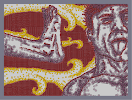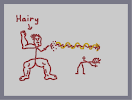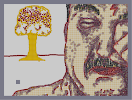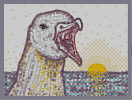Roundhouse To The Face The Attempt Transmorphers Beautiful Asian Woman Gull Celery: The Food of Champions

Pages: (2) [ 1 ] 2

FLAWLESS

### I would like this better

with harry potter.

### 5/5

I could tell you to stop bringing up this tiresome argument. Or I could take a nap.

### Stop

featuring maps with no featuring material. May the art be good, but featuring art is just ridiculous

### We had one two days ago njitsu

and the day before that. and the day before that. and the day before that and the day before that and the day before that and the day

### can we get a feature

that's playable and not a dda?

### much better

review than your last lovely n-art and review

### :D

You carved a niche into my heart.

No lie.

### Oh yeah

I remember this, I also remember trolling Espada under the guise of Kanye West.

### In my opinoin, it should be both, andreas

I mean, there isn't a finite amount of features.

### I look at Espada's stuff daily

lol.
Mmmm just as it used to appear in my mind xD
Is it just me who thinks a feature should go to an awesome but Overlooked map? this has 88 ratings and I really wouldn't call it overlooked.
Anyway gratz on the feature ;)

as I remembered

<3

### I love you, squibbles.

And this is absolutely going into my faves.

### Afraid I had to rush that review.

Just woke up, have to run.

Sorry that it's a little brief, and a lottle boring.

### Rater number

85! more rates than I have gotten in my life as of june 17. AWSOME!!!

### Wow

Awesome detail, and I love the shading. I wish the color didn't look so checkered, but all in all this is really nice. 5aved.

### No kidding

when I say this is the best N-art I have seen yet.

I voted for this for the dronies for you too :D
I <3 <3 <3 this/
5aved

### man

i just looked through some of your art and this is by far the best one. your a god at n art

### 5/5

It must be your best. I really loved it.

Totally epic.
Nice work

### ok then ;D

I'll plan one i guess ^^

its definetly between us three for the dronies. =]

### The checkered red and white made it look light red

Not pink! If you think this looks pink then you need you eyes fixed!

### @sondrigal

Checkered, what does red+white give you, pink.

### agree with flagmyidol

100%. it's beautiful, but some people just want to see a reason why it's not amazing. i can truely say that it is "flawless".

### When you want to have a color other than the ones given

You're forced to make the thumb ugly, there's no other way. It's hard to make a picture with splotches of mines+gold(to make orange) and door keys+gold(to make green) beautiful in full. (Or what ever color combination you want to use.)

...

### Techno

you get tingly sensations when looking at this? and I hope you spelled masterpiece like that on purpose. The checkered mines make a nice pink.
c'mon rt. it's pretty much impossible to make an n-art look good full and thumb. he's obviously didn't even try to make it a good full view, so don't dock him for it.

### This is really quite something.

Words cannot describe the tingly sensation that is currently rippling through various parts of my body.

Massterrrpeeace.

5/5aved

### eh

narts are the perennial over-rated. Yeah it looks great, but most narts look great. Plus in full view it looks terrible. This is an above average to very good nart at best.

### this is really beautiful

And I see it's back to 5/5 w/ 51 votes